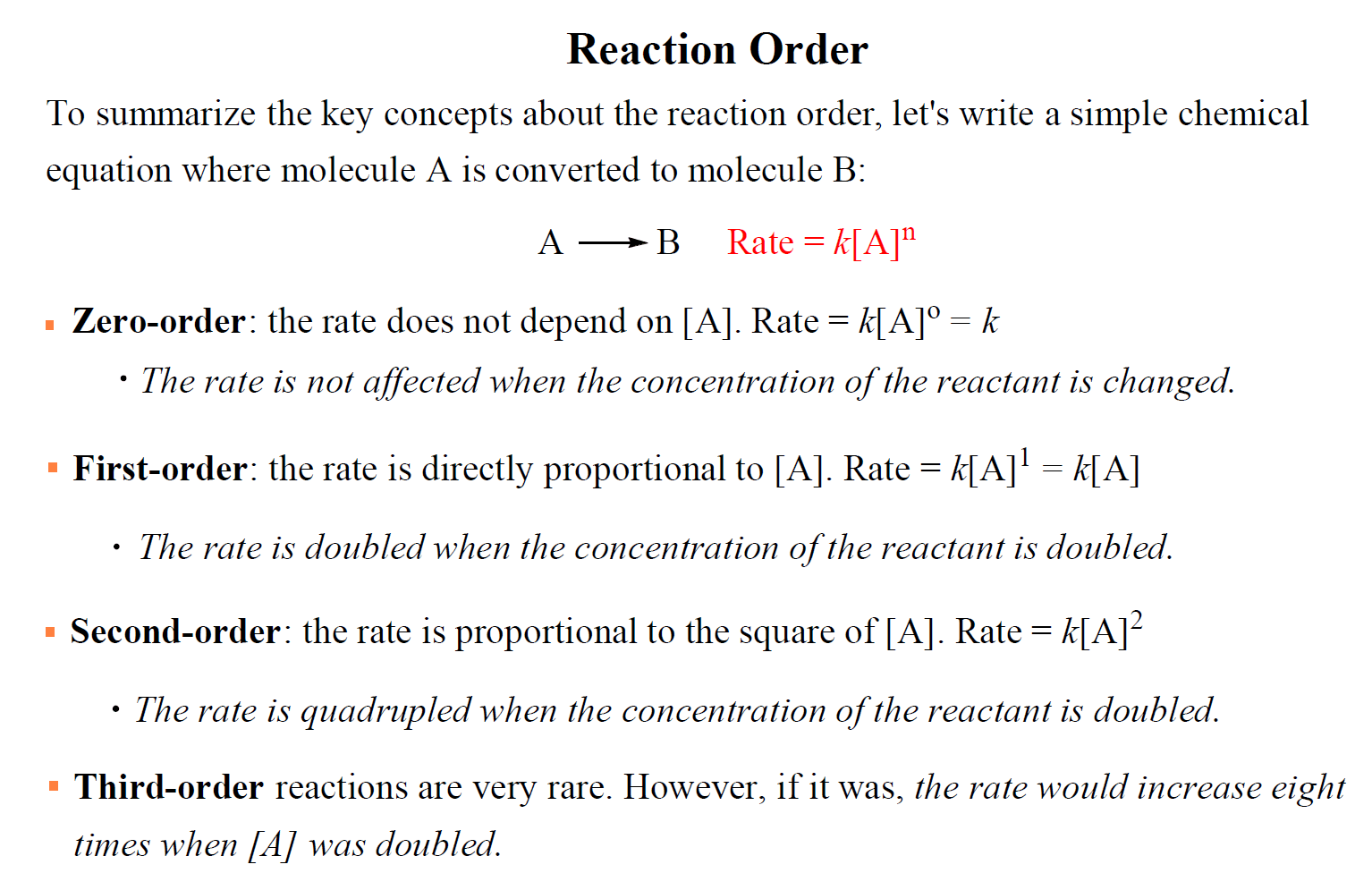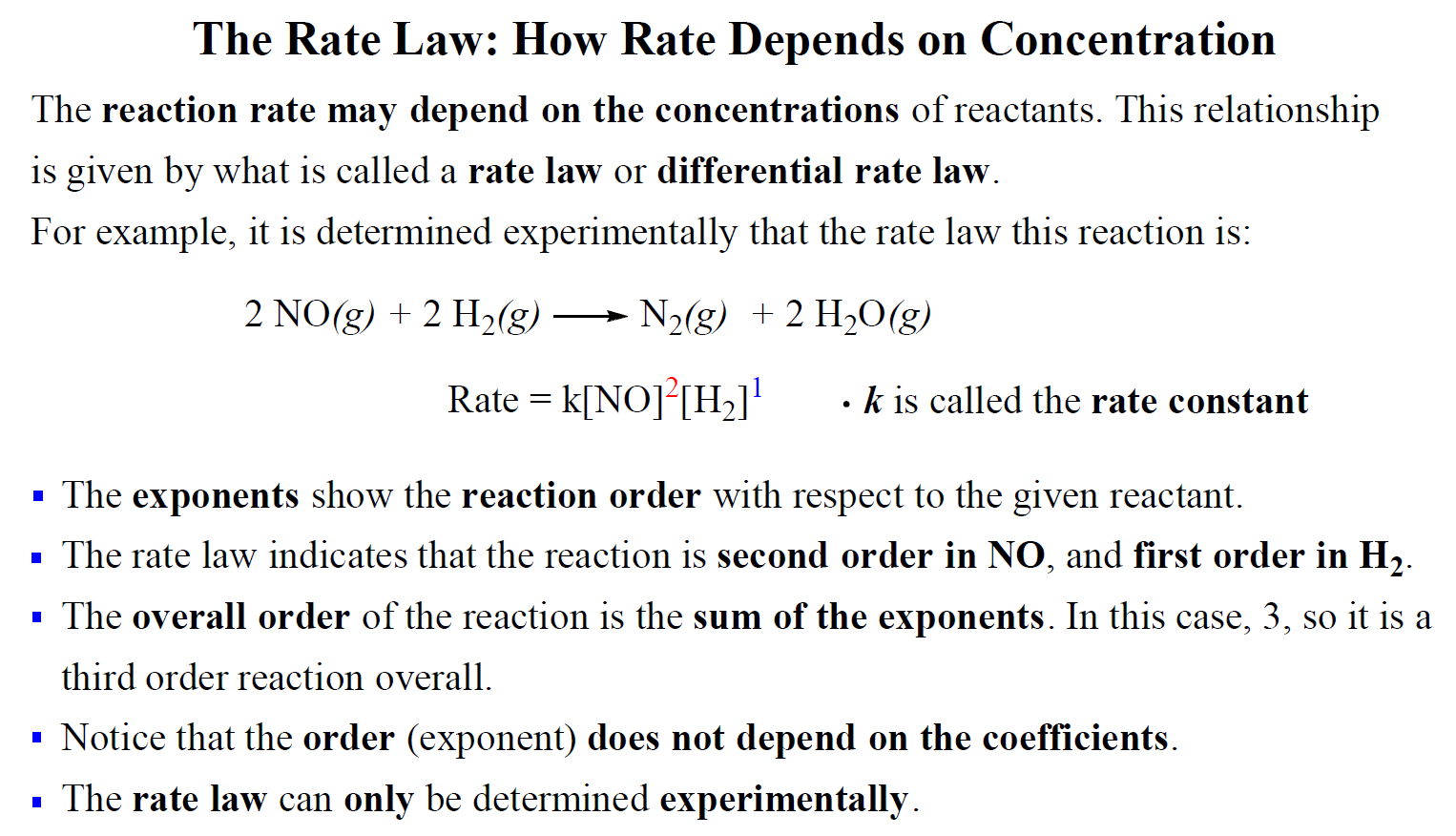## General Chemistry

In the previous post, we talked about the reaction rate and saw that it is not constant and changes with the concentration of reactants. This is not true for all reactions and it’s possible that the rate may be independent of the concentration.

How exactly the rate of a reaction depends on the concentration of reactant(s) is given by what is called the rate law or differential rate law of the reaction.

For a simple reaction with one reactant, the rate law could be written as:

A → Products

Rate = k[A]n

where k is the rate constant and n is the reaction order.

The rate constant is the proportionality constant between the reaction rate and the concentration of reactant(s).

The value of n is the reaction order, and it determines how the rate depends on the concentration of the reactant:

• If n = 0, the reaction is zero-order and the rate is independent of the concentration of A.
• If n = 1, the reaction is first order and the rate is directly proportional to the concentration of A.
• If n = 2, the reaction is second order and the rate is proportional to the square of the concentration of A.

For example, let’s say the concentration of A changes from 0.5 M to 0.3 M in a zero-order reaction. The rate for these two concentrations would be:

Rate = k[0.5]0 = k x 1= k

Rate = k[0.3]0 = k x 1= k

So, for a zero-order reaction, the rate is independent of reactant concentration is equal to the rate constant.

Let’s use 3 M and 6 M for the concentration of A and see how that affects the rate of a first-order reaction. The rates would be equal to:

Rate1 = k1 = 3k

Rate2 = k1 = 6k

The rate increases two times when we double the concertation of A. So, remember, in first-order reactions, the rate is directly proportional to the concentration of the reactant that appears in the rate law.

For a second-order reaction, the concentration of A is squared and therefore, the rate is proportional to the square of [A]:

Rate1 = k2 = 9k

Rate2 = k2 = 36k

$\frac{{{\rm{Rat}}{{\rm{e}}_{\rm{2}}}}}{{{\rm{Rat}}{{\rm{e}}_{\rm{1}}}}}\; = \;\frac{{36k}}{{9k}}\; = \;4$

So, doubling the concertation in a second-order reaction quadrupoles the reaction rate.

Third-order reactions are very rare. However, if it was, the rate would increase eight times when [A] was doubled

To summarize the key concepts about the reaction order, remember that:# How is the Reaction Order Determined?

It is important to know that the reaction order can only be determined experimentally. In most cases, the reaction order is not correlated to the coefficients of the reactants that appear in the rate law.

For example, the rate law of the following reaction was determined to be:

2NO(g) + 2H2(g)→ N2(g) + 2H2O(g)

Rate = k[NO]2[H2]1

Notice that there is a coefficient 2 in front of the hydrogen which does not transfer to the rate law.

For reactions when two reactants appear in the rate law, we mention the reaction order with respect to each reactant. In this case, the reaction is second-order with respect to NO and first-order in H2.

The overall order of the reaction is the sum of both exponents, and therefore, it is a third-order reaction overall.Here is a 77-question, Multiple-Choice Quiz on Chemical Kinetics:

Check Also